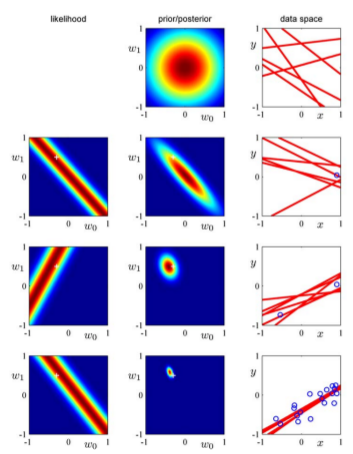# 基于贝叶斯估计的线性回归

## 2 参数分布

### 2.1 共轭先验估计

\begin{equation} \label{eq:1} p(\mathbf{w}) = \mathcal{N}(\mathbf{w}|\mathbf{m}_{0},\mathbf{S}_{0}) \end{equation}

### 2.2 后验估计

\begin{equation} \label{eq:2} p(\mathbf{w}|\mathbf{t}) = \mathcal{N}(\mathbf{w}|\mathbf{m}_{N},\mathbf{S}_{N}) \end{equation}

\begin{eqnarray} \label{eq:4} \mathbf{m}_{N}&=& \mathbf{S}_{N}(\mathbf{S}_{0}^{-1}\mathbf{m}_{0} + \beta \mathbf{\Phi}^{T} \mathbf{t}) \\ \mathbf{S}_{N}^{-1} &=& \mathbf{S}_{0}^{-1} + \beta \mathbf{\Phi}^{T}\mathbf{\Phi} \end{eqnarray}

## 3 一个例子

\begin{equation} \label{eq:5} p(\mathbf{w}|\alpha) = \mathcal{N}(\mathbf{w}|\mathbf{0},\alpha^{-1}\mathbf{I}) \end{equation}

\begin{eqnarray} \label{eq:7} \mathbf{m}_{N}&=&\beta \mathbf{S}_{N}\mathbf{\Phi}^{T}\mathbf{t} \\ \mathbf{S}_{N}^{-1}&=& \alpha \mathbf{I} + \beta \mathbf{\Phi}^{T}\Phi \end{eqnarray}

\begin{equation} \label{eq:8} \ln p(\mathbf{w}|\mathbf{t}) = -\frac{\beta}{2} \sum_{n=1}^{N} \{t_{n} - \mathbf{w}^{T}\mathbf{\phi}(\mathbf{x}_{n}) \}^{2} - \frac{\alpha}{2}\mathbf{w}^{T}\mathbf{w} + \mathrm{const} \end{equation}

1 演示了当数据量越来越多时先验估计和后验估计迭代更新的过程。Figure 1: 先验估计和后验估计迭代更新的过程

1 同时演示了当前的后验估计作为下一个数据到来时$$\mathbf{w}$$先验估计的过程。首先，第一行中间的图对应着还没有数据到达时$$\mathbf{w}$$域上的先验分布，最右边的图对应着随机取$$\mathbf{w}$$六次时$$y(\mathbf{w},x) = w_{0} + w_{1}x$$的图像。我们可以看到这个时候的图像完全是随机的，因为这个时候我们没有收到任何关于$$x$$的信息。

## 4 后记

\begin{equation} \label{eq:9} p(\mathbf{w}|\alpha) = \bigg[ \frac{q}{2} (\frac{\alpha}{2})^{1/q} \frac{1}{\Gamma(1/q)} \bigg]^{M}\exp\bigg( -\frac{\alpha}{2}\sum_{j=1}^{M}|w_{j}|^{q} \bigg) \end{equation}